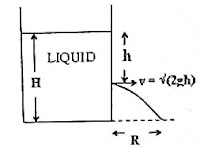## Pages

`“Life is like riding a bicycle.  To keep your balance you must keep moving.”–Albert Einstein`

## Monday, December 3, 2007

### Fluid Mechanics – Equations to be remembered

“There is no substitute for hard work.”
– Thomas A. Edison

You won’t be allowed to use equation tables and calculators to work out multiple choice questions (MCQ). (These are allowed in the case of free-response questions). You will have to remember certain basic formulas for solving multiple choice questions within the permitted time. The essential things you must remember in the section, ‘Fluid Mechanics’ are given below:
1. Weight of floating body = weight of displaced liquid
This can be modified as mass of floating body = mass of displaced fluid
2. Force of buoyancy (Fbuoy)= weight of displaced fluid
or, Fbuoy = Vρg where V is the volume of fluid displaced, ρ is the density of the fluid and g is the acceleration due to gravity.
3. Equation of continuity in fluid dynamics, as applied to liquids which are supposed to be incompressible, is A1v1= A2v2 where A1 and A2 are the cross section areas of the tube and v1 and v2 are the velocities of the liquid at these sections respectively.
4. Bernoulli’s equation (Bernoulli’s theorem) is
P + ρgh + (½)ρv2 = constant where P is the pressure, ρ is the density, ‘h’ is the height (with respect to the reference level for estimating the gravitational potential energy) and ‘v’ is the velocity of the fluid. The symbol ‘y’ also may be used in place of ‘h’.
Note that Bernoulli’s theorem follows from the law of conservation of energy in the case of a small mass ‘m’ of a fluid throughout its flow and can be written as
PV+ mgh + (½ )mv2 = constant
Another useful form of Bernoulli’s equation is
P/ρ + gh + (½)v2 = constant
In the case of a liquid flowing through a horizontal pipe (constant gravitational potential energy), the pressure of liquid will be smaller at points where the velocity is greater and vice versa.5.Torricelli’s Theorem:
Velocity of efflux (of liquid flowing out through a hole) =√(2gh)
where ‘h’ is the depth of the hole (fig).
(It is interesting to note that a particle dropped from a height ‘h’ will strike the ground with the above velocity)
The expression for the efflux velocity follows from Bernoulli’s theorem by considering the cases at the free surface of the liquid in the tank (fig) and at the hole:
P + ρgH + 0 = P + ρg(H–h) + (½)ρv2 so that v =√(2gh),
where P is the atmospheric pressure. Note that the hole is open to the atmosphere, as is the free liquid surface in the tank.
6. The horizontal range of liquid jet (fig), R= 2√[h(H–h)]
Note that the above expression is obtained by considering the horizontal range (on the ground) of a particle projected horizontally from a height (H–h). Do it as an exercise.
The range R will be maximum if h = H/2. [You may show this by putting dR/dh = 0 when R is maximum]. The maximum range is H.
In the next post we will consider some typical questions in this section. Of course, their solution also will be discussed.